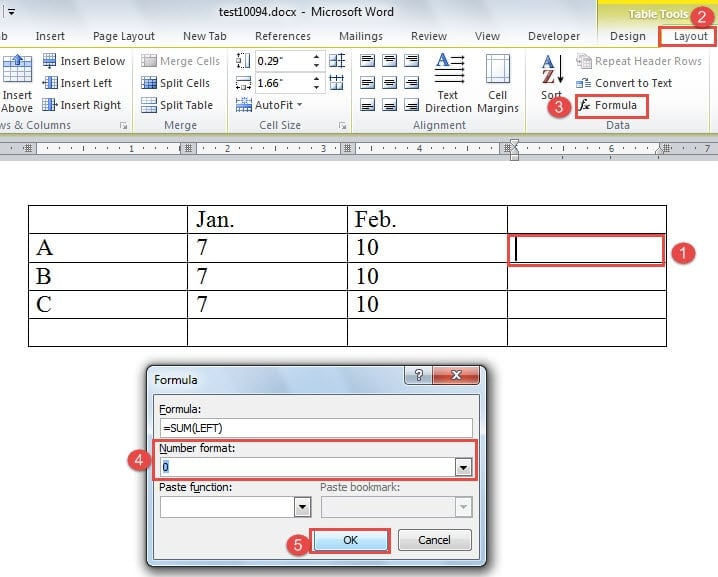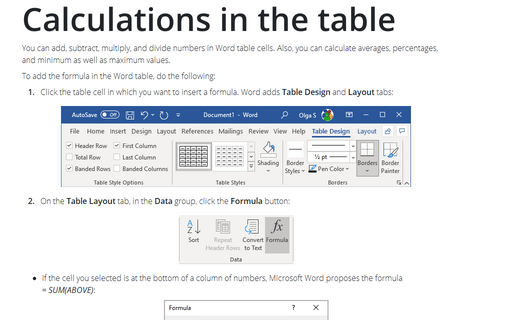# How To Add Formula In Word

### Learn to do basic calculations in Word tables.

When you think calculations you probably think first of Excel and that is as it should be. However, when the calculation is required inside a document, you might prefer to create a table in Word and then, have Word do the math for you. Word has a cut down collection of handy formulas for adding numbers and doing a range of calculations on table data.

I'll show you how to write formulas in Word tables to make calculations and and how to create a preformatted table and insert it into a document with one simple keystroke.

## Calculations in cells

You can do calculations in a Word table when the cells contain values or numbers. To do this, you need to know the table cell references - in other words the column letter and row number of the cell.

There is an old macro called TableCellHelper that you can still find and use that helps you do this. I wrote a post on it here and I have tested the instructions and it all works fine in all verions of Word up to and including Word 2013.

Basically the cells are labelled like a worksheet by the column number and row letter. The top left cell is A1, the one to its right is typically B1 and the one below it is A2. Where this numbering system comes crashing to a halt is when you have merged cells. However, with the TableCellHelper macro you can check a table cell if you're unsure - just click on the cell and run the macro.

Here are some typical table cell calculations that you might want to try out:

With one value in cell B2 and another in cell C2 you can total the result into cell D2. Click in cell D2, choose Table Tools > Layout > Formula.

Type =B2+C2 in the Formula area. Select the desired format from the Number format list and click Ok.

Understanding formulas

While you can type =B2+C2 in an Excel cell, you can't do the same thing in a Word table. A table formula must be entered using the Table Tools > Layout > Formula menu command or added as a Word field.

To see the field code that underlies a Word formula click on the formula and press Shift+F9.

It is possible to write Word formulas by hand if you wish to do so. Click in the cell and press Control + F9 to add the field code braces. Now type the formula into the cell, for example, this calculates the percentage of the value in D2 that the value in C2 represents and formats the result as a percentage:

= 100*C2/D2 # '0%'

When you're done, press Shift+F9 to see the result.

Recalculating formulas

Unlike an Excel worksheet, Word formulas don't automatically recalculate so, when you change the value in a table, any formulas which use that value will show an incorrect result unless and until you force a recalculation.

The same will be the case if you had a cell showing an Error messages - the error won't disappear until you force a recalculation to be made.

To force the recalculation, click in the formula's result or in the error so you have the underlying field code targetted and press F9.

Summing a column

To total a column of values in a Word table, click in the cell that should contain the answer, choose Table Tools > Layout > Formula and type the formula =sum(above).

Referencing cells

It is possible to reference a cell in a table outside the table. To do so you must first create a bookmark for the value by selecting the entire field code in the table and choose Insert > Bookmark > Name, type a name and click Add.

To refer to the value in your text, click at the point to include the value and choose Insert > Quick Parts > Field and in the Field names list choose Ref and select the bookmark name you created.